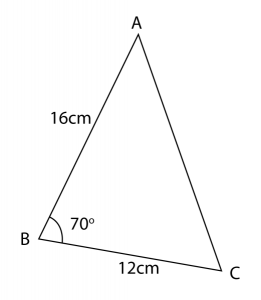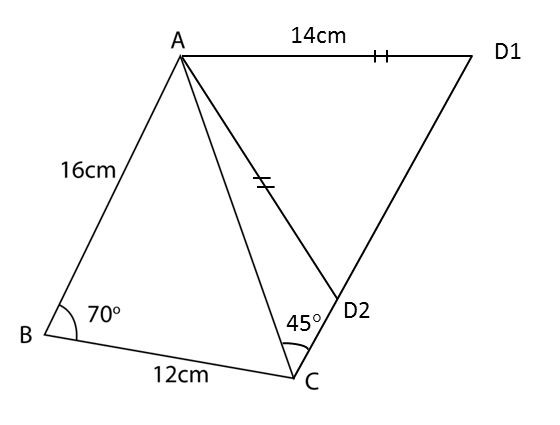\

# 9.4.3 Solution of Triangles Long Questions (Question 5 & 6)

Question 5:
The diagram below shows a triangle ABC.(a) Calculate the length, in cm, of AC.
(b) A quadrilateral ABCD is now formed so that AC is a diagonal, ACD = 45° and AD = 14 cm. Calculate the two possible values of ADC.
(c) By using the acute ADC from (b), calculate
(i) the length, in cm, of CD,
(ii) the area, in cm2, of the quadrilateral ABCD

Solution:
(a)
Using cosine rule,
AC2 = AB2 + BC2 – 2 (AB)(BC) ABC
AC2 = 162 + 122 – 2 (16)(12) cos 70o
AC2 = 400 – 131.33
AC2 = 268.67
AC = 16.39 cm

(b)(c)(i)

(c)(ii)

Question 6:
Diagram below shows trapezium ABCD.(a) Calculate
(i) ∠BAC.
(ii) the length, in cm, of AD.
(b) The straight line AB is extended to B’ such that BC = B’C.
(i) Sketch the trapezium AB’CD.
(ii) Calculate the area, in cm2, of ∆BB’C.

Solution:
(a)(i)

(a)(ii)

(b)(i)(b)(ii)